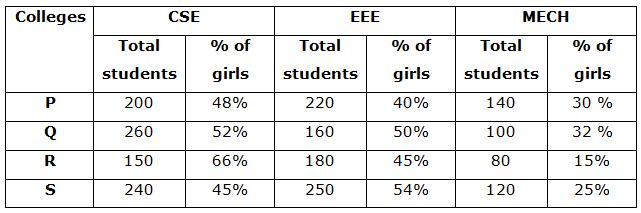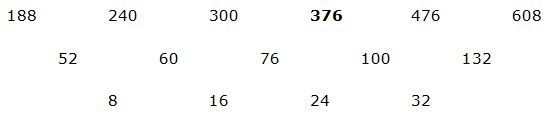# CWC/FCI Prelims 2019 – Quantitative Aptitude Questions (Day-43)

Dear Readers, Exam Race for the Year 2019 has already started, To enrich your preparation here we are providing new series of Practice Questions on Quantitative Aptitude – Section for CWC/FCI Exam. Aspirants, practice these questions on a regular basis to improve your score in aptitude section. Start your effective preparation from the right beginning to get success in upcoming CWC/FCI Exam.

[WpProQuiz 5951]

Directions (Q. 1 – 5): Find the wrong term in the following number of series?

1) 72, 37, 36, 55, 109, 273, 819.5

a) 72

b) 37

c) 109

d) 273

e) 819.5

2) 188, 240, 300, 374, 476, 608

a) 374

b) 476

c) 188

d) 240

e) 608

3) 573, 571, 580, 552, 616, 491

a) 571

b) 491

c) 616

d) 580

e) 552

4) 11, 15, 37, 133, 557, 2821

a) 15

b) 37

c) 133

d) 557

e) 11

5) 3150, 1575, 525, 131.5, 26.25

a) 1575

b) 3150

c) 26.25

d) 525

e) 131.5

Directions (Q. 6 – 10): Study the following information carefully and answer the given questions:

The following table shows the total number of students studied in various branches of four different engineering colleges and the percentage of girls among them.6) Find the difference between the total number of boys studied in CSE department from college P and S together to that of total number of girls studied in MECH department from college Q and R together?

a) 152

b) 192

c) 134

d) 116

e) None of these

7) Find the average number of girls studied in EEE department from all the given colleges together?

a) 96

b) 102

c) 88

d) 104

e) None of these

8) Find the ratio between the total number of boys studied in EEE department from college R to that of total number of girls studied in MECH department from college S?

a) 18 : 7

b) 25 : 11

c) 72 : 55

d) 33 : 10

e) None of these

9) Find the total number of girls studied in all the given departments from college S?

a) 384

b) 273

c) 328

d) 245

e) None of these

10) Total number of students studied in college P is approximately what percentage of total number of students studied in college R in all the given department together?

a) 122 %

b) 105 %

c) 137 %

d) 85 %

e) 156 %

Directions (1-5):

The correct series is,

72, 37, 36, 55, 109, 273.5, 819.5

The pattern is, *0.5 + 1, *1 – 1, *1.5 + 1, *2 – 1, *2.5 + 1, *3 – 1,…

The wrong term is, 273

The correct series is,The difference of difference is, 8, 16, 24, 32,….

The wrong term is, 374

The correct series is,

573, 571, 580, 552, 617, 491

The pattern is, -(13 +1), +(23 +1), -(33 +1), +(43+1), -(53 +1),….

The wrong term is, 616

The correct series is,

11, 15, 39, 133, 557, 2821

The pattern is, *1 + 22, *2 + 32, *3 + 42, *4 + 52, *5 + 62,…..

The wrong term is, 37

The correct series is,

3150, 1575, 525, 131.25, 26.25

The pattern is, ÷ 2, ÷ 3, ÷ 4, ÷ 5,….

The wrong term is, 131.5

Directions (6-10):

The total number of boys studied in CSE department from college P and S together

= > 200*(52/100) + 240*(55/100)

= > 104 + 132 = 236

The total number of girls studied in MECH department from college Q and R together

= > 100*(32/100) + 80*(15/100)

= > 32 + 12 = 44

Required difference = 236 – 44 = 192

The total number of girls studied in EEE department from all the given colleges together

= > 220*(40/100) + 160*(50/100) + 180*(45/100) + 250*(54/100)

= > 88 + 80 + 81 + 135 = 384

Required average = 384/4 = 96

The total number of boys studied in EEE department from college R

= > 180*(55/100)

The total number of girls studied in MECH department from college S

= > 120*(25/100)

Required ratio = [180*(55/100)] : [120*(25/100)]

= > 33: 10

The total number of girls studied in all the given departments from college S

= > 240*(45/100) + 250*(54/100) + 120*(25/100)

= > 108 + 135 + 30 = 273

Total number of students studied in college P in all the given department together

= > 200 + 220 + 140 = 560

Total number of students studied in college R in all the given department together

= > 150 + 180 + 80 = 410

Required % = (560/410)*100 = 136.585 % = 137 %